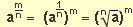## Test Questions

### Try some of the following to test your skills:

1. 73 x 7 = 9.
 23 x (22)4 = 25
2. 56 x 52 = 10. 64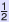=
3. 95 ÷ 90 = 11. 2−3 =
4. 35 ÷ 3-2 = 12. 5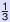5.
 10 x 103 = 102
13. 4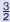6. (43)4 = 14.
 2−1 + ( 1 )= 16
7. 72 x 78 x 73 = 15. 9÷ 88. 312 x 35 ÷ 34 =

1. 74 9. 27
2. 58 10. 8
3. 95 11.
 1 8
4. 37 12.
 1 5
5. 102 13.
 1 8
6. 47 14.
 3 4
7. 713 15. 31
8. 313

### Remember the rules of indices:

Multiplying powers with the same base     am x an = am+n

Dividing powers with the same base         am ÷ an = am−n

Raising a power to a power                     (am)n = amn

Raising any number to the power zero     a0= 1   (also a1= a)

 Negative powers and reciprocals a−m   = 1 (a−m is the reciprocal of am) am

Fractional powers and roots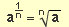and